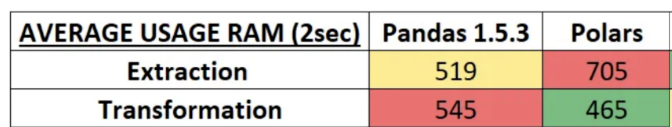• Pandas 2.0正式版发布： Pandas vs Polars速度对比
• 发布于 1个月前
• 99 热度
0 评论
Pandas 2.0正式版在4月3日已经发布了，以后我们pip install默认安装的就是2.0版了，Polars 是最近比较火的一个DataFrame 库，最近在kaggle上经常使用，所以这里我们将对比下 Pandas 1.5，Polars，Pandas 2.0 。看看在速度上 Pandas 2.0有没有优势。

Polars
Polars 是一个 Rust 和 Python 中的快速多线程 DataFrame 库/内存查询引擎。它使用 Apache Arrow作为内存模型在 Rust 中实现。它在2021年3月发布。

Polars的一些主要特点如下：

Polars的一个最大好处是，它不仅有Python的包，Nodejs，Rust等也可以方便的进行继承使用，并且经过各方的验证，它的确要比Pandas1.x快很多。

Pandas 2.0

https://pandas.pydata.org/docs/dev/whatsnew/v2.0.0.html

``` !wget https://github.com/DataTalksClub/nyc-tlc-data/releases/download/yellow/yellow_tripdata_2021-01.csv.gz
!wget https://d37ci6vzurychx.cloudfront.net/trip-data/yellow_tripdata_2021-01.parquet```

` !wget https://s3.amazonaws.com/nyc-tlc/misc/taxi+_zone_lookup.csv`
1、提取

pandas
``` def pd_read_csv(path, engine_pd,):
"""
Converting csv file into Pandas dataframe
"""
return df
"""
Converting parquet file into Pandas dataframe
"""
return df```
Polars
``` def pl_read_csv(path, ):
"""
Converting csv file into Pandas dataframe
"""
return df
"""
Converting parquet file into Pandas dataframe
"""
return df```

``` path1="yellow_tripdata_2021-01.csv.gz"
path2="taxi+_zone_lookup.csv"
path1="yellow_tripdata_2021-01.parquet"
path2 = "taxi+_zone_lookup.csv"
2、转换

Pandas代码：
``` def mean_test_speed_pd(df_pd):
"""
Getting Mean per PULocationID
"""
df_pd = df_pd[['PULocationID', 'trip_distance']]
df_pd["PULocationID_column"] = df_pd[['PULocationID']].astype(int)
df_pd=df_pd.groupby('PULocationID').mean()
return df_pd

def endwith_test_speed_pd(df_pd):
"""
Only getting Zones that end with East
"""

df_pd = df_pd[df_pd.Zone.str.endswith('East')]

return df_pd
Polars
def mean_test_speed_pl(df_pl):
"""
Getting Mean per PULocationID
"""
df_pl = df_pl[['PULocationID', 'trip_distance']].groupby('PULocationID').mean()
return df_pl

def endwith_test_speed_pd(df_pl):
"""
Only getting Zones that end with East
"""

df_pl = df_pl.filter(pl.col("Zone").str.ends_with('East'))

return df_pl```
3、加载

pandas
``` def loading_into_parquet(df_pd, engine):
"""
Save dataframe in parquet
"""
df_pd.to_parquet(f'yellow_tripdata_2021-01_pd_v{pd.__version__}.parquet',engine)
polars
"""
Save dataframe in parquet
"""
df_pl.write_parquet(f'yellow_tripdata_2021-01_pl.parquet')```
4、结果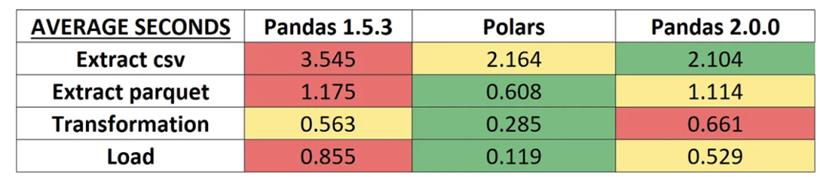CPU和RAM分析

``` import os
import psutil

def process_memory():
process = psutil.Process(os.getpid())
mem_info = process.memory_info()

def process_cpu():
"""
Getting cpu_percent in last 2 seconds
"""
cpu_usage = psutil.cpu_percent(2)
return cpu_usage

# decorator function mem
def profile_mem(func):
def wrapper(*args, **kwargs):

mem_before = process_memory()
result = func(*args, **kwargs)
mem_after = process_memory()
print("Consumed memory: {:,}".format(
mem_before, mem_after, mem_after - mem_before))

return result
return wrapper

# decorator function cpu
def profile_cpu(func):
def wrapper(*args, **kwargs):
result = func(*args, **kwargs)
cpu_after = process_cpu()
print(f"Consumed cpu: {cpu_after}")

return result
return wrapper```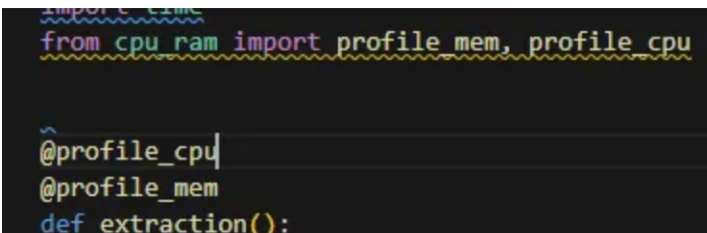parquet文件提取的新脚本，最终的时间结果与前面测试类似：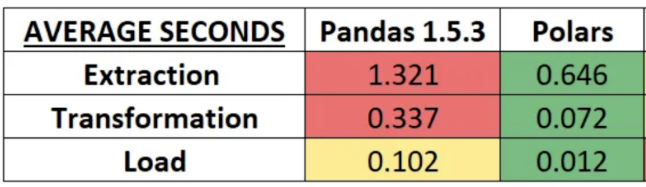CPU结果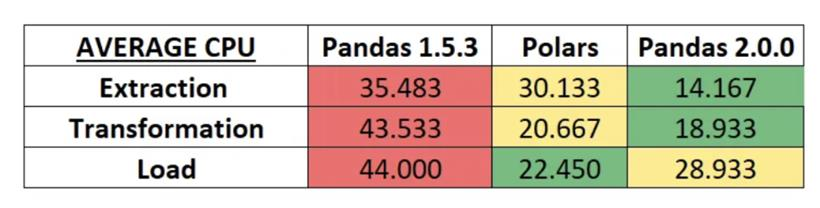RAM的结果